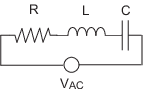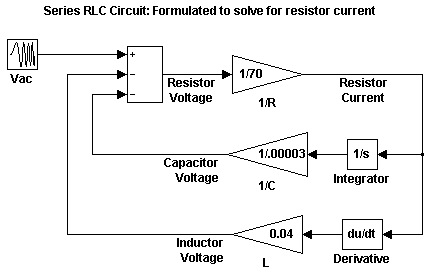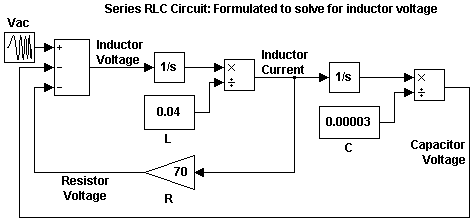## 最佳形式的数学模型

### 串联 RLC 示例`${V}_{AC}={V}_{R}+{V}_{L}+{V}_{C}$`

`${V}_{AC}=Ri+L\frac{di}{dt}+\frac{1}{C}{\int }_{-\infty }^{t}i\left(t\right)dt$`

### 使用电阻电压解算串联 RLC

`$\begin{array}{l}{V}_{R}=Ri\\ Ri={V}_{AC}-L\frac{di}{dt}-\frac{1}{C}{\int }_{-\infty }^{t}i\left(t\right)dt\end{array}$`

#### 电路模型### 使用电感电压解算串联 RLC

`$\begin{array}{l}{V}_{L}=L\frac{di}{dt}\\ L\frac{di}{dt}={V}_{AC}-Ri-\frac{1}{C}{\int }_{-\infty }^{t}i\left(t\right)dt\end{array}$`

#### 电路模型